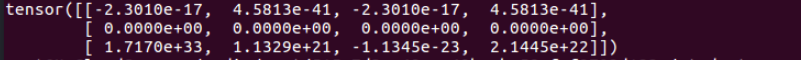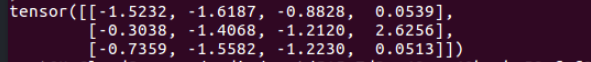# Pytorch：权重初始化

## 1、不初始化的效果

w = torch.Tensor(3,4)
print (w)## 2、初始化的效果

PyTorch提供了多种参数初始化函数：

w = torch.Tensor(3,4)
torch.nn.init.normal_(w)
print (w)## 3、初始化神经网络的参数

class Net(nn.Module):

def __init__(self, block, layers, num_classes=1000):
self.inplanes = 64
super(Net, self).__init__()
***
*** #定义自己的网络层
***

for m in self.modules():
if isinstance(m, nn.Conv2d):
n = m.kernel_size * m.kernel_size * m.out_channels
m.weight.data.normal_(0, math.sqrt(2. / n))
elif isinstance(m, nn.BatchNorm2d):
m.weight.data.fill_(1)
m.bias.data.zero_()

***
*** #定义后续的函数
***


def weights_init(m):
classname = m.__class__.__name__
if classname.find('Conv2d') != -1:
init.xavier_normal_(m.weight.data)
init.constant_(m.bias.data, 0.0)
elif classname.find('Linear') != -1:
init.xavier_normal_(m.weight.data)
init.constant_(m.bias.data, 0.0)


model = Net(*****)
model.apply(weights_init)